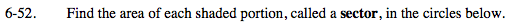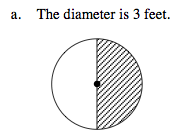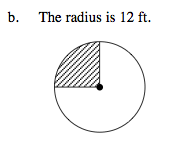### Home > MC2 > Chapter 6 > Lesson 6.1.5 > Problem6-52

6-52.Refer to Problem 6-41 for help.The radius is half the diameter.

Therefore the radius is 1.5 feet. Now use the equation for the area of a circle: A=r2π

Substitute 1.5 for r.
A=(1.5)2π

The area of the entire circle is approximately 7 square feet. Since only half of the circle is shaded, we must divide the total area by 2.

3.5 square feetFind the area of the circle, then divide it by 4 since
only one-fourth of the circle is shaded.